# Quiz Discussion

If P(A)=2/5​, P(B)=3/10​ and P(A ∩ B) =51​, then P(A' | B'). P(B' | A') is equal to

Course Name: Quantitative Aptitude

• 1]

5/6

• 2]

5/7

• 3]

25/42

• 4]

1

##### Solution
No Solution Present Yet

#### Top 5 Similar Quiz - Based On AI&ML

Quiz Recommendation System API Link - https://fresherbell-quiz-api.herokuapp.com/fresherbell_quiz_api

# Quiz
1
Discuss

What is the sum of all 4 digit numbers that can be formed by the digits 1, 2, 3, 4 each exactly once

• 1]

55566

• 2]

66660

• 3]

56456

• 4]

84738

##### Solution
2
Discuss

The number of triangles that can be formed by choosing the vertices from a set of 12 points, seven of which lie on the same straight line, is

• 1]

185

• 2]

175

• 3]

115

• 4]

105

##### Solution
3
Discuss

Three unbiased coins are tossed. What is the probability of getting at most two heads ?

• 1]

3/4

• 2]

1/4

• 3]

3/8

• 4]

7/8

##### Solution
4
Discuss

How many integers between 1000 and 10000 have no digits other than 4, 5, or 6?

• 1]

91

• 2]

71

• 3]

51

• 4]

81

##### Solution
5
Discuss

There are 8 orators A, B, C, D, E, F, G, and H. In how many ways can the arrangements be made so that A always comes before B and B always comes before C.

• 1]

8! / 3!

• 2]

8! / 6!

• 3]

5! x 3!

• 4]

8! / (5! x 3!)

##### Solution
6
Discuss

A biased coin in tossed thrice. What is the probability that heads turns out at least twice considering that the probability of a head is 60%?

• 1]

0.648

• 2]

0.234

• 3]

0.348

• 4]

.839

##### Solution
7
Discuss

If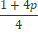,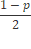​,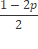are probabilities of three mutually exclusive events, then

• 1]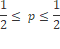• 2]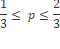• 3]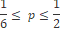• 4]

None Of These

##### Solution

8
Discuss

In how many ways can 10 people line up at a ticket window of a railway station?

• 1]

36,28,800

• 2]

34,82,800

• 3]

33,44,800

• 4]

33,28,800

##### Solution
9
Discuss

A person tosses an unbiased coin. When head turns up, he gets Rs.8 and tail turns up he loses Rs.4. If 3 coins are tossed, what is probability that he gets a profit of Rs.12?

• 1]

3/8

• 2]

5/8

• 3]

3/4

• 4]

1/8

##### Solution

Person will get profit of Rs 12 only when there is 2H (Head) and 1T (Tail)

H + H + T = 12

8 + 8 + (-4) = 12

Total outcome of 2 head and 1 tail = 23 = 8

i.e (T, H, TH, HT, HH, HHT, HTH, THH)

Total event with 2H and 1 T is 3

therfore probability = 3/8

10
Discuss

In a simultaneous throw of two dice, what is the probability of getting a total of 7?

• 1]

1/6

• 2]

1/4

• 3]

2/3

• 4]

3/4

# Quiz